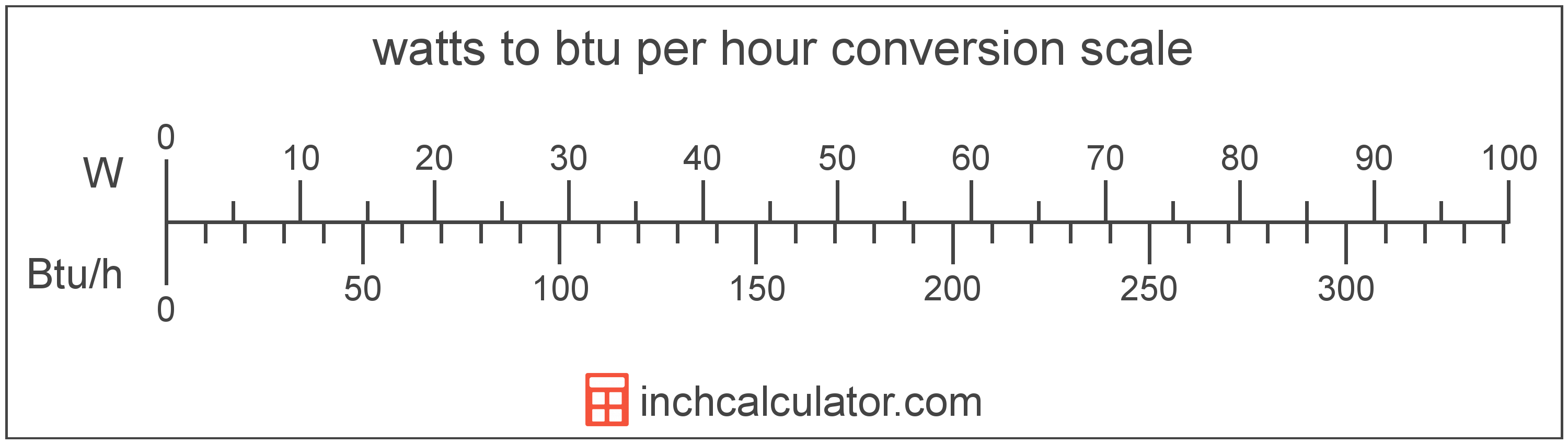# Btu per Hour to Watts Converter

Enter the power in btu per hour below to get the value converted to watts.

Results in Watts:1 Btu/h = 0.293071 W

Do you want to convert watts to btu per hour?

## How to Convert Btu per Hour to Watts

To convert a measurement in btu per hour to a measurement in watts, multiply the power by the following conversion ratio: 0.293071 watts/btu per hour.

Since one btu per hour is equal to 0.293071 watts, you can use this simple formula to convert:

watts = btu per hour × 0.293071

The power in watts is equal to the power in btu per hour multiplied by 0.293071.

For example, here's how to convert 5 btu per hour to watts using the formula above.
watts = (5 Btu/h × 0.293071) = 1.465355 W### How Many Watts Are in One Btu per Hour?

There are 0.293071 watts in one btu per hour, which is why we use this value in the formula above.

1 Btu/h = 0.293071 W

## What Are Btu per Hour?

One BTU per hour is equal to one british thermal unit, or the energy needed to heat one pound of water 1 °F, for one hour.

The btu per hour is a US customary unit of power. Btu per hour can be abbreviated as Btu/h; for example, 1 btu per hour can be written as 1 Btu/h.

In the expressions of units, the slash, or solidus (/), is used to express a change in one or more units relative to a change in one or more other units.

## What Is a Watt?

One watt is the power equal to the rate of energy of one joule per second in an electrical circuit. One watt is also equal to the power produced in a circuit with a voltage potential of one volt with a current of one amp.

The watt is the SI derived unit for power in the metric system. Watts can be abbreviated as W; for example, 1 watt can be written as 1 W.

Watt's Law states the relationship between power, current, and voltage. Using Watt's Law, it's possible to express the power in watts as an expression using current and voltage.

PW = IA × VV

The power in watts is equal to the current in amperes times the potential difference in volts.

Combining this with Ohm's Law, it's also possible to express the power in watts using resistance in addition to volts and amps.

## Btu per Hour to Watt Conversion Table

Table showing various btu per hour measurements converted to watts.
Btu Per Hour Watts
1 Btu/h 0.293071 W
2 Btu/h 0.586142 W
3 Btu/h 0.879213 W
4 Btu/h 1.1723 W
5 Btu/h 1.4654 W
6 Btu/h 1.7584 W
7 Btu/h 2.0515 W
8 Btu/h 2.3446 W
9 Btu/h 2.6376 W
10 Btu/h 2.9307 W
11 Btu/h 3.2238 W
12 Btu/h 3.5169 W
13 Btu/h 3.8099 W
14 Btu/h 4.103 W
15 Btu/h 4.3961 W
16 Btu/h 4.6891 W
17 Btu/h 4.9822 W
18 Btu/h 5.2753 W
19 Btu/h 5.5683 W
20 Btu/h 5.8614 W
21 Btu/h 6.1545 W
22 Btu/h 6.4476 W
23 Btu/h 6.7406 W
24 Btu/h 7.0337 W
25 Btu/h 7.3268 W
26 Btu/h 7.6198 W
27 Btu/h 7.9129 W
28 Btu/h 8.206 W
29 Btu/h 8.4991 W
30 Btu/h 8.7921 W
31 Btu/h 9.0852 W
32 Btu/h 9.3783 W
33 Btu/h 9.6713 W
34 Btu/h 9.9644 W
35 Btu/h 10.26 W
36 Btu/h 10.55 W
37 Btu/h 10.84 W
38 Btu/h 11.14 W
39 Btu/h 11.43 W
40 Btu/h 11.72 W

## References

1. International Bureau of Weights and Measures, The International System of Units, 9th Edition, 2019, https://www.bipm.org/documents/20126/41483022/SI-Brochure-9-EN.pdf